## DUOTONEMAP

 Enhances the shadows and/or highlight regions in an image.

### EXAMPLES

 Example 1 Original Arguments: defaults redist 40,70,70 -auto-level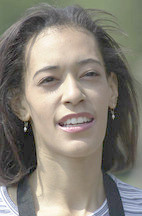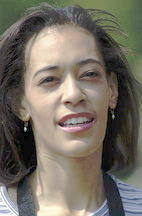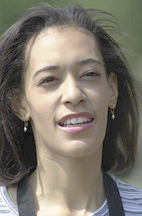Example 2 Original redist 50,70,70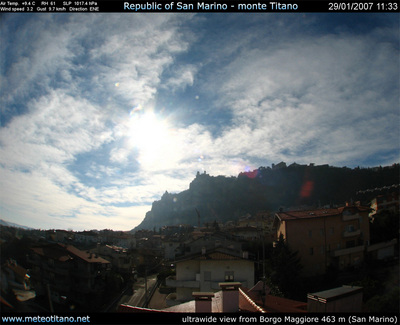Arguments: -m both (default) Arguments: -m shadowExample 3 Original redist 50,50,40Arguments: -m both (default) Arguments: -m shadow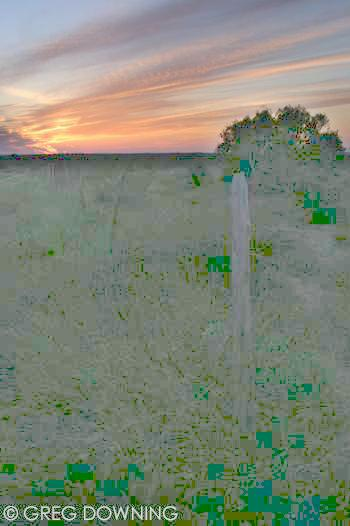Arguments: -m both -s 4 -h 1 Arguments: -m both -s 6 -h 1.1Example 4 Original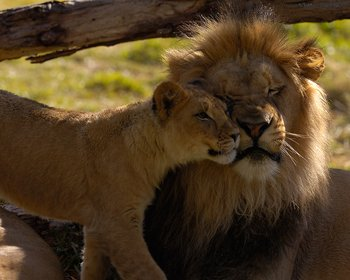Arguments: (default) redist 60,60,60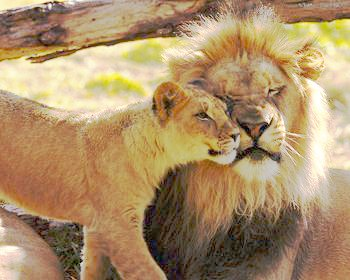What the script does is as follows: Computes the mean of the image Thresholds the image at the mean Blurs the thresholded image over the ramp distance Applies the mask to the image and computes the mean and gamma value for the highlights Applies the negated mask to the image and computes the mean and gamma value for the shadows Applies the highlight gamma to the input image Applies the shadow gamma to the inputimage Composites the two gamma modified images using the mask image to generate the output This is equivalent to the following IM commands thresh=`convert \$infile -format "%[fx:100*(mean+\$bias/100)]" info:` convert \$infile -threshold \${thresh}% -blur \${ramp}x65000 \$tmpA2 meanm=`convert \$tmpA2 -format "%[fx:mean]" info:` means=`convert \$infile \( \$tmpA2 -negate \) -compose multiply -composite \ -format "%[fx:mean/(1-\$meanm)]" info:` meanh=`convert \$tmpA1 \$tmpA2 -compose multiply -composite \ -format "%[fx:mean/\$meanm]" info:` sgamma=`convert xc: -format "%[fx:log(\$means)/log(\$lower)]" info:` hgamma=`convert xc: -format "%[fx:log(\$meanh)/log(\$upper)]" info:` convert \$infile -auto-level -gamma \$sgamma \$tmpA3 convert \$infile -auto-level -gamma \$hgamma \$tmpA4 convert \$tmpA3 \$tmpA4 \$tmpA2 -compose over -composite \$outfile